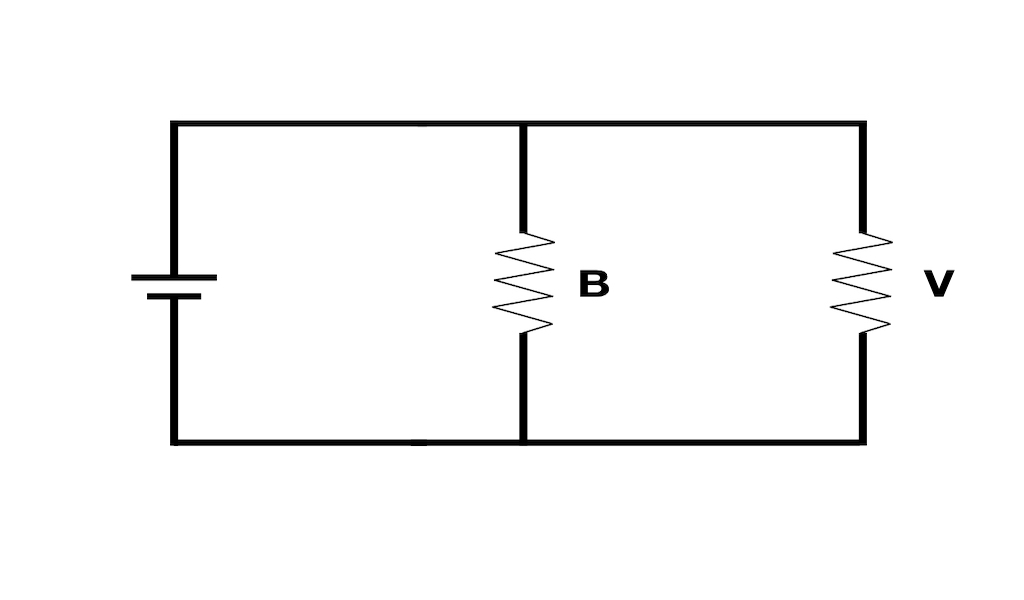# The bias-variance circuit under g-priorsThe bias-variance tradeoff is usually discussed in terms of the mean squared error (MSE) of a predictor. However, it can also be applied to estimates of coefficients in a linear model. Below we examine how bias and variance figure into the MSE of coefficient estimates under a $$g$$-prior.

Assume a linear model of the form

$Y = X \beta + \epsilon$

with independent errors $$\epsilon_i \sim \mathcal{N}(0, \sigma^2)$$. Then

$Y \sim \mathcal{N}\Big(X \beta, I_n / \phi\Big)$

where $$\phi = 1/\sigma^2$$ is the inverse noise variance.

In general, the MSE of an estimator $$\hat{\beta}$$ decomposes neatly into

$\mathsf{E}\big[||\beta - \hat{\beta}||_2^2\big] = \text{tr}\Big( \Sigma_{\hat{\beta}} \Big) + \mathsf{E} \Big[ (\beta - \hat{\beta}) \Big]^T \mathsf{E} \Big[ (\beta - \hat{\beta}) \Big]$

which is a multivariate analog of the traditional bias-variance decomposition. The first term involving $$\Sigma_{\hat{\beta}}$$ – the covariance matrix of $$\hat{\beta}$$ – is the variance component of the error, while the second term is the bias component of the error.

In ordinary least squares (OLS) estimation, $$\hat{\beta}$$ is unbiased, so the error it incurs will be entirely due to the variance component above:

$\text{MSE}_{\text{OLS}} = \frac{1}{\phi} \text{tr}\Big( (X^T X)^{-1} \Big)$

However, we can do better if we are willing to inject some prior information into the model. In Bayesian inference, a common way to do this is to put something called a $$g$$-prior on the coefficients $$\beta$$:

$\beta | \phi, g \sim \mathcal{N}\Big(0, \frac{g}{\phi} (X^T X)^{-1} \Big)$

which yields a posterior distribution on the coefficients of the form

$\beta | \phi, g, Y \sim \mathcal{N}\Big(\frac{g}{1+g} \hat{\beta}_\text{OLS}, \frac{g}{1+g} \frac{1}{\phi} (X^T X)^{-1} \Big)$

From the parameterization above, we can see that as $$g$$ gets large, the posterior mean of the coefficients gets close to the OLS estimator for the coefficients. Since the OLS estimator is unbiased, its expectation is $$\beta$$ – the true coefficients – which means the posterior mean is unbiased in the limit as $$g \rightarrow \infty$$.

However, for $$g < \infty$$, the posterior mean under the $$g$$-prior is not unbiased. In fact, its bias is given by

$\mathsf{E}_{Y | \beta, g}\big[\beta - \hat{\beta}_\text{post} \big] = \frac{1}{1+g} \beta$

which yields the following decomposition for MSE:

\begin{aligned} \mathsf{E}_{Y|\beta, \phi, g}\big[||\beta - \hat{\beta}_\text{post}||_2^2\big] = \Big(\frac{g}{1+g}\Big)^2 \bigg[\frac{1}{\phi}\text{tr}\Big((X^TX)^{-1}\Big)\bigg] + \Big(\frac{1}{1+g}\Big)^2 \bigg[\beta^T\beta\bigg] \end{aligned}

Note that the above expression is again a weighted sum of variance ($$V$$) and bias ($$B$$) components with

\begin{aligned} V &:= \frac{1}{\phi}\text{tr}\Big((X^TX)^{-1}\Big) \\ B &:= \beta^T\beta \end{aligned}

Under a $$g$$-prior, then, we know that we will incur some error due to the variance of our estimator and some error due to the squared magnitude of the true coefficients. The question then becomes: can we pick a value of $$g$$ that minimizes the weighted sum of these components?

In practice, the answer is no because the “right” value of $$g$$ will depend on unknown quantities like $$\phi$$ and the true coefficients $$\beta$$. In theory, however, we can solve for the minimizer to get

$g_\text{min} = \frac{B}{V}$

yielding an MSE of

$\text{MSE}_{g_{\min}} = \frac{VB}{V+B}$

Physics students might quickly recognize the formula above as a “product over sum,” which describes the total resistance in a circuit with resistors in parallel. The connection here may be purely coincidental, but it does lead to a couple of interesting analogies:

#### Optimal wiring

• Stats Q:
• Given two sources of error, $$B$$ and $$V$$, and a weighting of each component parameterized by $$g$$, what is the minimal MSE we can obtain?
• Physics Q:
• Given two resistors of resistance $$B$$ and $$V$$, respectively, what is the total resistance under the minimal-resistance wiring?
• Answer for both: $$\frac{VB}{V+B}$$

#### Domination of Bayesian estimator under optimal wiring

• Stats Q:
• If the minimizing $$g$$ could be found, would the MSE for the posterior mean under the $$g$$-prior always be less than or equal to the MSE under OLS?
• Physics Q:
• Suppose we have two circuits, one with a single resistor of resistance $$V$$, the other with a resistor of resistance $$V$$ and a resistor of resistance $$B$$, wired in parallel. Will the total resistance of the second always – no matter what the value of $$B$$ is – be less than or equal to the total resistance of the first?
• Answer for both: yes, since $$V \cdot \frac{B}{V+B} \leq V \cdot 1 = V$$

Since the author is not a physicist, it will take some extra work to determine whether the analogies above can be extended further, or whether more exist. It would be nice to examine whether the $$g$$-priors themselves come with any special physical intuition that informs this picture of resistors in parallel.